# 117. Create a Formula

 < Day Day Up >

Calc makes it easy to add simple formulas in your spreadsheet.

##Before You Begin

116 About Creating Formulas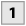Start Formula

Select the cell that will contain the formula. Type an equal sign (=) or select the Function button (the big equal sign on the Formula bar).Select Cell

Select the first cell that will be included in the formula (selecting the cell is better than typing the cell address because it avoids typos). The cell address appears in the cell where you are creating the formula.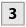Enter Operator and Select Second Cell

Type the operator for the formula. For example, type an asterisk (*) for multiplication. Then select the second cell that will be acted on by the formula. Press Enter to complete the formula. The results of the formula appear in the cell where you built the formula. To view the formula itself, select the cell and view the formula on the Formula bar's Input line.

Tip

After you create the formula, you can use the autofill feature to copy the formula to other cells or use Copy and Paste to copy the formula to other cells.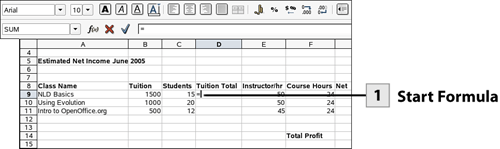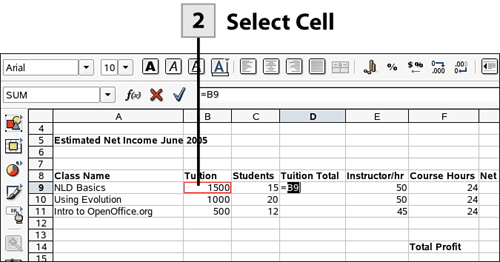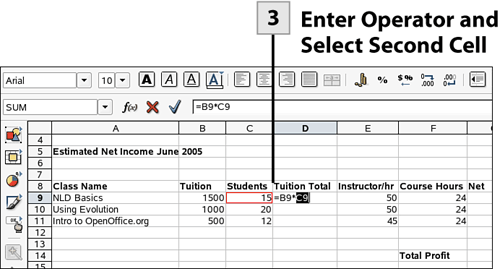< Day Day Up >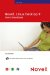Novell Linux Desktop 9 Users Handbook
ISBN: 0672327295
EAN: 2147483647
Year: 2003
Pages: 244
Authors: Joe Habraken

flylib.com © 2008-2017.
If you may any questions please contact us: flylib@qtcs.net